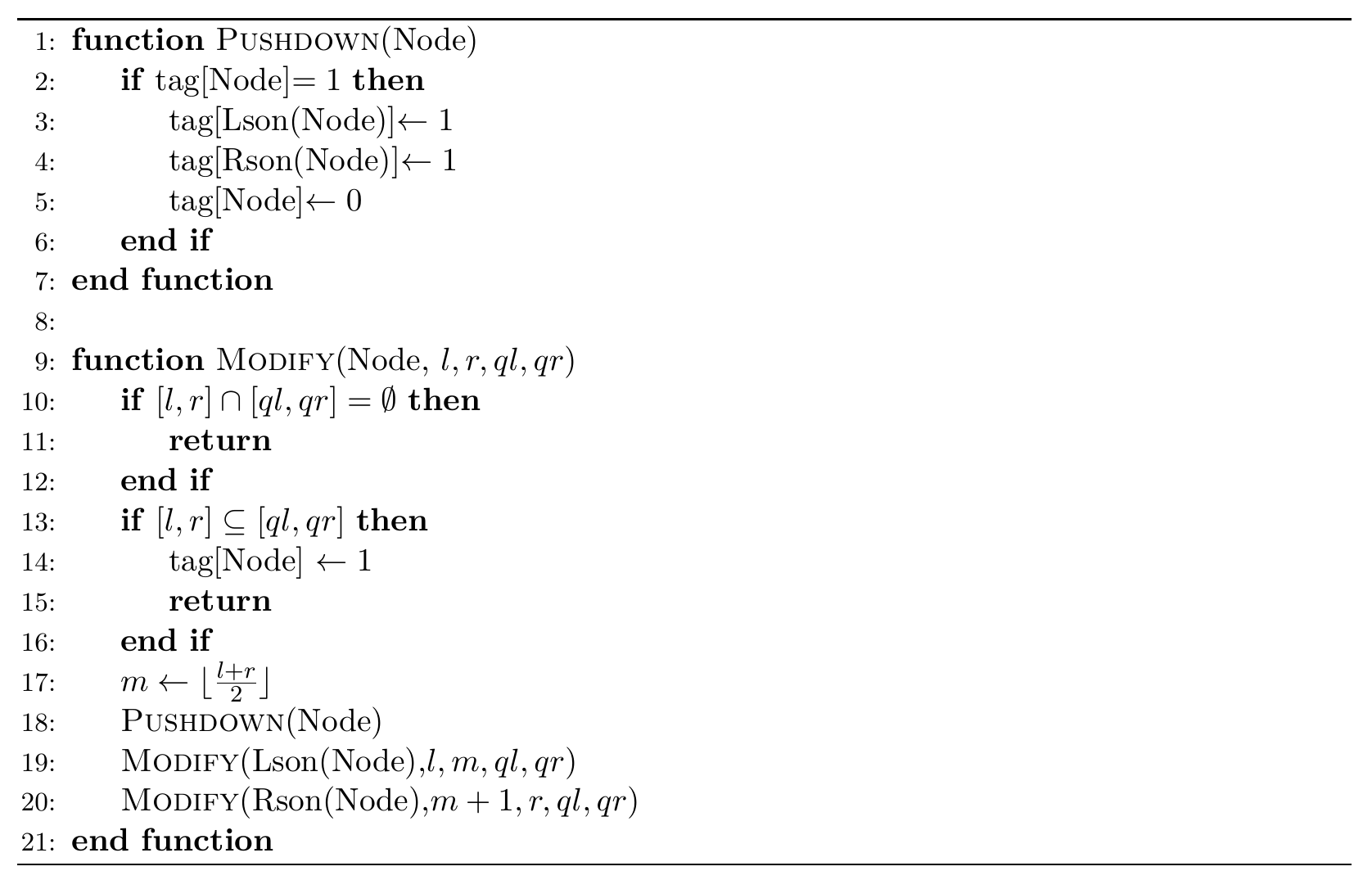# #585. 【ZJOI2020】传统艺能

Bob 喜欢线段树。

Bob 有一棵根为 $[1, n]$ 的广义线段树。Bob 需要在这个线段树上执行 $k$ 次区间懒标记操作，每次操作会等概率地从 $[1, n]$ 的所有 $\frac{n(n+1)}2$ 个子区间中随机选择一个。对于所有在该次操作中被访问到的非叶子节点，Bob 会将这个点上的标记下推；而对于所有叶子节点（即没有继续递 归的节点），Bob 会给这个点打上标记。

Bob 想知道，$k$ 次操作之后，有标记的节点的期望数量是多少。

#### 具体定义### 样例一

#### input

3 1
1 2



#### output

166374060



### 样例二

#### input

5 4
2 1 3 4



#### output

320443836



### 限制与约定

$1$ $\le 10$ $\le 4$
$2$ $\le 10$ $\le 100$
$3$ $\le 5$ $\leq10^9$
$4$ $\leq 200000$ $=1$
$5$ $=32$ $\leq10^9$ 输入的线段树为完全二叉树
$6$ $=64$
$7$ $=4096$
$8$ $\le 5000$ 每个 $m$ 均在 $[l, r-1]$ 内均匀随机
$9$ $\le 100000$
$10$ $\leq 200000$